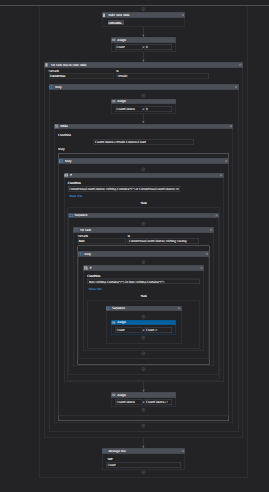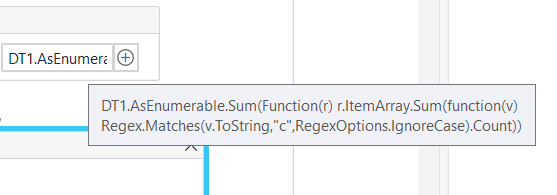# How to count character in excel using uipath

here i want to count C in total spreadsheet how to be anyone help me thanks

Welcome to UiPath community

Can you tell us more detail on this and share the Output

From my understanding You need to calculate the Character C in the excel file right?

Regards
Gokul

yes i want to count how many c in total excel sheet

Check out the Updated XAML fileZeroInTheString.xaml (15.1 KB)

Regards
Gokul

We assume that you already have Read the Excel sheet as Datatable and have the datatable variable, say `DT`. Then we could check with the below query.

``````TotalCCount = (From r In DT
Let cCount = r.ItemArray.Sum(Function(x)x.ToString.Count(Function(y)y.ToString.ToLower.Equals("c")))
Select cCount).Sum
``````

Here, `DT` is your datatable variable and `TotalCCount` is an Integer variable which holds the total count of the character c

Alternately or maybe a better method is to use `Output Datatable` Activity and use Regex to find the total number of occurrences of the character c.

``````System.Text.RegularExpressions.Regex.Matches(strDT.ToLower,"c").Count
``````

Removed - due parallel worked result was similar to Arpans @supermanPunch suggestions